# Basic Principles

This section includes basic background information on the theory of electrochemical detection. It is not mathematically oriented. Concepts important to the LCEC technique are explained. Bioanalytical Systems commercialized these concepts in 1974. While the instrumentation has evolved a great deal since then, the basic principles remain the same.

## ELECTROCHEMICAL FUNDAMENTALS

LCEC may be considered in terms of electrolysis at a fixed point along a flowing stream. The stream in this case is the eluent from the analytical LC column and a sequence of solute ("analyte") zones separated with varying degrees of resolution. These zones pass into a very low-volume thin-layer cell, where the flow is constrained to a thin film passing over a planar electrode held at a fixed potential. Figure 1.1 illustrates an exploded view of this region. If the potential is greater (more positive for oxidation, more negative for reduction) than that required for the electrolysis of the analyte, a measurable charge passes from electrode to analyte (or vice versa). The resulting current is directly proportional to the concentration of solute passing through the channel.

Figure 1.1. Exploded view of electron transfer at the surface of a thin-layer electrode. A laminar flow profile passes over the electrode in the thin-layer zone containing reduced analyte R. Oxidation to O at the electrode surface releases electron(s) to the surface. This current is subsequently converted to a voltage, which drives the recorder and produces the chromatogram.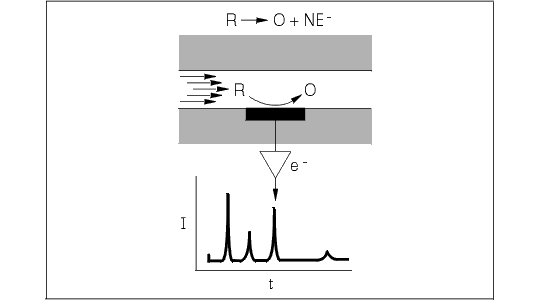The electrode may be thought of as a chemical reagent. The more positive its potential, the stronger an oxidizing agent it becomes; when the potential is made more negative, it becomes a stronger reducing agent. In either case, as the concentration of solute rises and falls in passing through the thin-layer cell, the electrolysis current proportionately follows these changes. This current, as a function of time, is amplified and sent to a recorder to yield a chromatogram.

LCEC is an AMPEROMETRIC determination. Unlike measurements (such as pH) of a potential difference under zero-current conditions, LCEC measures current at a fixed potential. It must always be remembered that this experiment involves heterogeneous electron transfer (from one phase to a different phase), and the success of the experiment depends in large part upon the care with which these components are chosen and operated.

Crucial to all amperometric determinations is FARADAY'S LAW, which states that:

(1) Q = nFN

Q is the number of coulombs (a unit of charge) used in converting N moles of material, n is the number of moles of electrons lost or gained in the transfer process per mole of material, and F is Faraday's constant (96,500 coulombs/mole of electrons). Differentiation of (1) with respect to time yields the current, which is the measure of the rate at which material is converted:

(2) dQ/dt = i = nF dN/dt

Equation (2) therefore relates a measurable quantity, the current, to the fundamental redox process occurring in the cell.

Bioanalytical Systems established a new standard for its cross-flow electrochemical transducers in the BASi 200A and BASi 480 liquid chromatography systems (Figure 1.2). As from the beginning, the proven thin-layer concept remains central to this design. In it, two blocks form a sandwich around a thin fluoropolymer gasket which defines a microliter flowcell. Two working electrodes (glassy carbon or other material) are embedded along one wall of the channel, whereas the reference and auxiliary electrodes are directly opposite, typically only 50 micrometers distant.

Figure 1.2. Cross-flow thin layer cell for LCEC.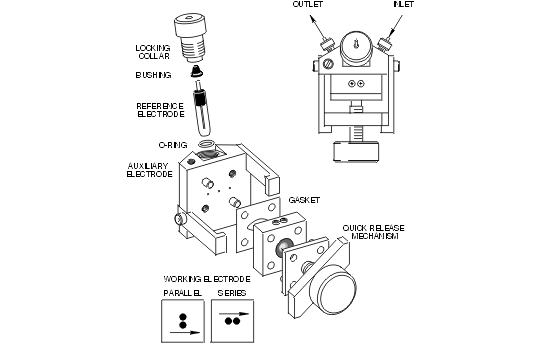In 1994, BASi introduced the UniJet cell (Figure 1.3), specifically for microbore LCEC. The UniJet utilizes a radial flow thin-layer cell which has some advantages in low-flow, low-dead-volume situations.

Figure 1.3. UniJet cell design for BASi microbore chromatography.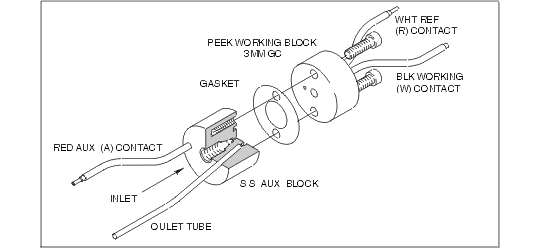The nature of the working electrode, particularly its bulk composition and surface treatment, is critical to detector performance. Many organic compounds react at significantly different rates depending on the electrode used. It is normally desirable to carry out the electrode reactions at the greatest possible rate in order that the current be limited only by mass transport of molecules to the surface and not by their reaction rate at the surface. This situation affords the greatest sensitivity and stability without sacrificing selectivity.

Carbon paste (a mixture of spectroscopic graphite powder and a dielectric material such as mineral oil, silicone oil, and paraffin wax) was used for initial LCEC experiments (ca. 1972) due to its excellent properties for organic electrochemical reactions. For most published applications of carbon paste electrodes, the useful lifetime of the electrode surface can extend to many months. However, if extremes of potential are used (> 1 V vs. Ag/AgCl) and/or if the mobile phase contains high concentrations of organic solvent, the electrode surface must be renewed more frequently. Carbon paste is rarely used now, since most users prefer the convenience of glassy carbon. However, it is still hard to match the performance of a well-packed carbon paste electrode.

Glassy carbon falls more closely in line with our desire for a universal electrode material. Glassy carbon is a hard, amorphous carbon material capable of being polished to a mirror-like finish. When housed in a PEEK block, a glassy carbon cell offers good solvent resistance, a feature particularly useful with mobile phases containing acetonitrile or large percentages of methanol. It has been used in entirely nonaqueous systems with good success.

It is widely believed that sample molecules, lipids, or proteins will "poison" the electrode surface after a few samples have been injected. This notion undoubtedly arises from classical voltammetry (including polarography), where the presence of molecules of high molecular weight leads to serious problems. Voltammetric measurements quite often involve sample concentrations over the range 10-5 to 10-3 moles/liter. In some cases, the electrode reaction itself leads to polymer formation, which "passivates" the surface, causing unreliable results.

In the case of amperometric detection, the column isolates the electrode from many contaminants, the mobile phase continuously cleanses the surface, and the usual sample concentrations are in the range of 10-8 to 10-6 moles/liter. Furthermore, the electrode is only exposed to individual compounds for a minute or less due to the narrow elution zones encountered in liquid chromatography. All of these factors heavily favor the LC electrode in comparison with direct (i.e., no chromatography) electrochemical measurements. Hence, with LCEC experiments, electrode "life" is extended much longer than with traditional voltammetric experiments.

The LC-4C and LC-3C detectors set the reference electrode at ground potential and the working potential in reference to this. The epsilon system sets the working electrode to ground potential and the reference electrode at -E. This situation often baffles those who have not been introduced to the simple fact that it is the POTENTIAL DIFFERENCE BETWEEN AN ELECTRODE AND THE SOLUTION which is of importance in electrochemistry and NOT the potential of the electrode material itself. Normally, this potential difference extends across an interphase region (the "electrical double layer") which is quite thin. It is the purpose of the electronics to control this potential difference while at the same time converting the current (resulting from the electrode reaction) into a voltage which is easily recorded or processed by a computer.

Figure 1.4 illustrates a recorder tracing encountered following initial application of a predetermined potential difference to a thin-layer cell containing a freshly prepared carbon paste electrode. The mobile phase is passing though the detector cell, but no sample has been injected into the chromatograph. Why then is so much current measured? Initially, some charge is required for the electrode/solution interface to achieve the applied potential difference. Additional charge will pass as the oxidation states of functional groups on the graphite are altered to a new state of equilibrium. Finally, impurities in the mobile phase and the mobile phase itself will oxidize (or reduce) resulting in a steady-state background current. Water will oxidize (or reduce) at all potentials although the rate of the reaction is quite slow except at very positive (or negative) extremes.

Figure 1.4. Typical equilibration response of electrode when it is first turned on.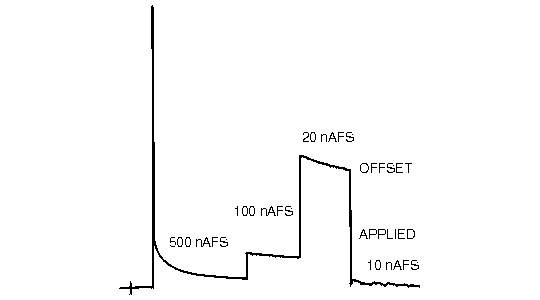The background current is electronically canceled by the offset current applied by the user. This results in a steady baseline at recorder zero (Figure 1.4). It is always desirable to operate with the minimum absolute background current to optimize sensitivity. The chromatogram is recorded "on top" of a current which often exceeds the peak heights for eluted compounds. High background currents increase the susceptibility of the instrument to noise (e.g., pump pulsations) and can result in serious nonlinear (negative) deviations in calibration curves.

Figure 1.5. Performance parameters for detector evaluation.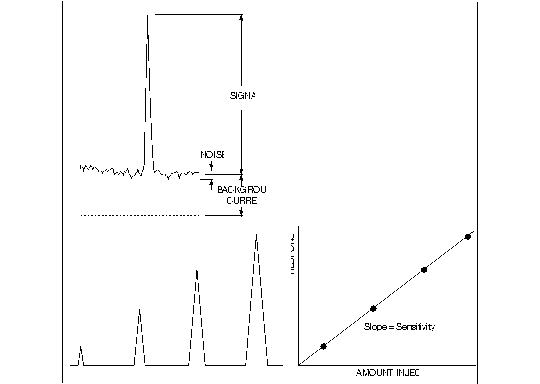It is useful to evaluate the peak-to-peak baseline noise over a time period about ten times the width of the chromatographic peak (Figure 1.5). The frequency content of the noise relative to frequencies much higher or lower can usually be removed. The high frequencies are easily filtered out and the very low frequencies are, in effect, baseline drift which is eliminated when the peak is quantitated. The amount injected that would give a peak height equal to the background current noise is clearly below the limit of usefulness. An amount that would give a peak height five times the background current noise is useful under many circumstances.

Figure 1.5 summarizes in graphic form the various parameters useful in evaluating detector performance. These are often confused in real practice. Sensitivity is the slope of the response versus amount. Detection limits refer to the amount of analyte required to give a signal X times greater than the noise (usually X = 3). The two terms are not synonymous. The reader should be able to foresee situations in which two detectors demonstrate equal sensitivity but unequal detection limits. The only difference necessary is the noise. We recommend that LCEC users report sensitivity for each peak of interest as well as the noise. With this information, it is possible for the reader to predict a reasonable detection limit for each substance being determined.

## HYDRODYNAMIC VOLTAMMOGRAMS

Let's begin our discussion by using a hypothetical electrochemical detector. Suppose we are operating the unit in an oxidative mode with a carbon electrode, and have initially chosen a potential low enough so that no detection of our test analyte should be possible. An injection of the test analyte is made; as predicted, no response is seen at the expected time. The potential is raised another 100 mV, the injection repeated, and the current response noted. This process is repeated until the potential becomes so high that the background current is prohibitive (usually +1.2 V). Suppose our test analyte were electroactive in the range examined. Then a plot of peak height versus applied potential would look similar to the solid line in Figure 1.6B.

Figure 1.6. Hydrodynamic voltammogram for mobile phase alone (A) and solute in mobile phase (B). The waveform for the solute is characterized by the half-wave potential E1/2.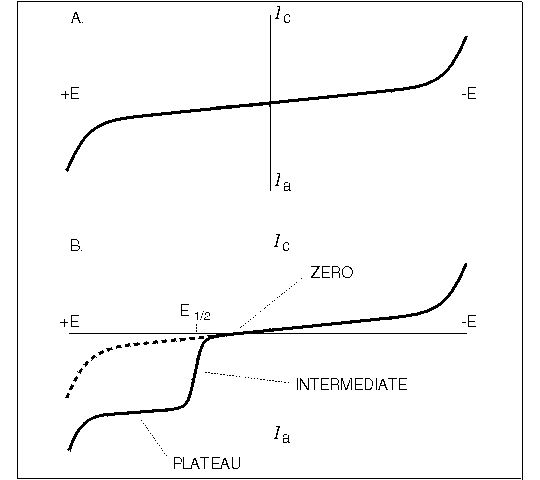Three zones along this curve would become apparent:

1. Zero current region. The potential was not sufficient to force oxidation to occur.

2. Intermediate region. The peak height is rising with increasing potential. Here the potential is controlling the kinetics of the heterogeneous electron transfer from the solute to the electrode surface.

3. Plateau region. In this zone, the peak height is independent of the potential. Diffusion to the electrode surface is the rate-determining factor; that is, the current is proportional to the rate of transport of molecules per unit surface area and per unit time.

In most situations, it is advantageous to operate the detector at a potential in the plateau region within 50-100 mV of the final break in the curve. Such a choice will offer maximum selectivity. The background current will be minimal and fewer interferences are likely. These advantages diminish when high potentials (> 1 V) are required. This point is illustrated in Figure 1.7 where the voltammograms are shown for equimolar solutions of four oxidizable compounds (A-D).

Figure 1.7. Left: Hypothetical hydrodynamic voltammograms for four oxidizable solutes A, B, C, and D. Right: Hypothetical chromatograms for EC detector operated at potentials 1-5.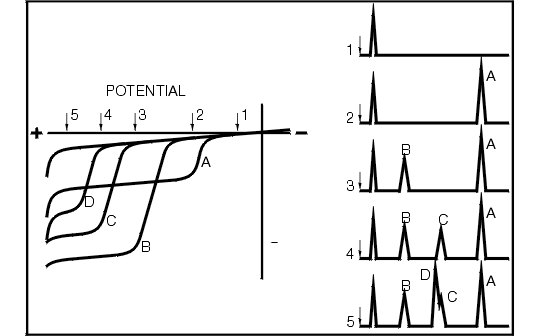The curves have different oxidation potentials, as exemplified by the wave locations. Each compound also has its own particular limiting current, depending on such factors as the number of electrons transferred, the rate of that transfer from bulk solution to the surface, and the type of electrode surface used. Substance A, for example, is easier to oxidize than C, but yields a greater current response due to some combination of the above factors. Detecting A is very simple via LCEC. By setting the potential at 2, A may be clearly measured with no response from B, C, or D. In order to detect C, however, the potential must be set at a value which is sufficient for oxidation of B and A as well. Selectivity in this case is also dependent on the chromatography. Figure 1.7 also illustrates the chromatograms that would be obtained for potentials 1 through 5. Note that once in a plateau region, the current response for that compound is the same for any higher potential. Also, the farther one moves out in potential, the greater the number of compounds that will show up on a complex chromatogram. At potential 5, the selectivity (both electrochemically and chromatographically) is inadequate to permit separation of C and D.

Dual-electrode LCEC can overcome some of the limitations described above. Since BASi dual electrodes are interchangeable between parallel and series arrangements, and since different electrode materials can be used together in one cell, several alternatives not available in single-electrode cells become possible. In the previous example, it might be possible to distinguish between C and D based on a difference measurement in a dual series configuration. Detailed examples of the use of dual electrodes for complex problems are available in BAS Application Capsules

## OXIDATION OR REDUCTION?

Most of the discussion thus far assumes that you have some idea of whether you will be looking at either an oxidative or a reductive electrochemical reaction. What if you don't know? Don't panic! It is not always obvious. Hydrodynamic voltammograms can give you some information, but they require several injections onto your LCEC analyzer, use mobile phase, and put wear on the column. There is an easier way.

Cyclic voltammetry (CV) is a quick and inexpensive means of learning the electrochemical behavior of the analyte in the mobile phase you plan to use. Mobile phase changes can cause shifts in applied potential. To keep the assay optimized, you should either do an HDV as described, or run a cyclic voltammogram on the sample in your new mobile phase. A sample cyclic voltammogram is illustrated in Figure 1.8. CV data for a wide range of compounds can be found here. If you are continually faced with new analytical problems, you will find a CV instrument an invaluable accessory for LCEC.

Figure 1.8. This cyclic voltammogram of thyronine tells us that a potential of +0.85-0.90 V is necessary to detect it. It also undergoes an interesting follow-up reaction which could be detected by dual-electrode LCEC.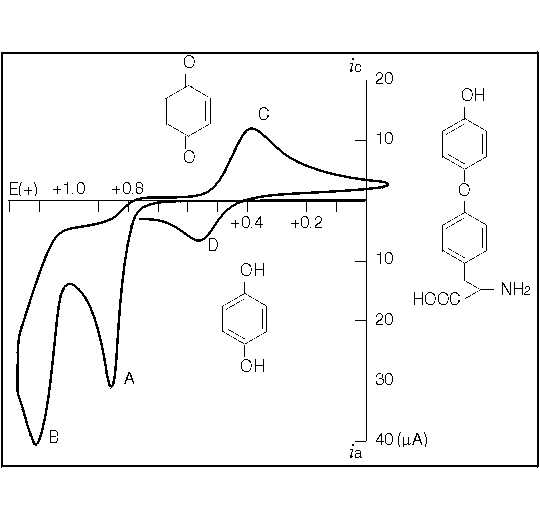## REFERENCE ELECTRODES

The role of the reference electrode in any electrochemical cell is to provide a stable half-cell potential. Recall the Nernst equation, which states that the half-cell potential, E, is a function of the thermodynamic E°, measured at unit activity for all species involved, and the various activities of the components of the half-cell reaction.

E = E° + (RT/nF)logK

K is the equilibrium constant for the half-cell reaction, written as an oxidation.

For the Ag/AgCl reference electrode commonly used in LCEC detection, the reaction and corresponding Nernst equation are as follows:

Ag° + Cl- â†’ AgCl + e-

E = E° + 0.059 log (1/[Cl-])

The activities of the Ag wire and AgCl are unity and, therefore, to a good first approximation, only the chloride ion concentration determines the E value.

What affects the stability of the E value? From our experience, any minor drift in reference electrode E is usually due to a change in Cl- The concentration inside BASi reference electrodes is initially 3 M. Since the chloride ion concentration in the mobile phase is typically zero, a steep concentration gradient exists along the porous frit (at the tip) separating these two solutions. Since the concentration gradient allows the chloride ion concentration to decrease by continuous diffusion into the mobile phase, the potential must necessarily change as dictated by the Nernst equation. Although other chloride concentrations could be used with a reference electrode half cell, the problem of the concentration gradient would still exist, and drift in the reference electrode potential would likewise occur.

On a time scale of several weeks, the potential of the reference electrode half cell may drift to values on the order of 40 to 50 mV from when the reference electrode was originally installed in the chromatograph. On a short-term basis, this type of drift is not objectionable, because the change per day would only amount to a few millivolts. Since the applied potential required to force the LCEC oxidation or reduction to occur is generally set at least 20 to 50 mV in excess of that required, the detector's response factors are maintained at an adequate level. In isolated cases, however, the reference electrode may experience catastrophic failure. This degree of failure is indicated by the magnitude of drift in the reference electrode's potential, and the error normally amounts to +0.5 to +1.0 V. Obviously the reference electrode is not providing a stable potential. Usually, the cause of such deterioration is mechanical, and the reference electrode will require replacement.

In terms of caring for reference electrodes, a few guidelines are important. First, when using a reference electrode, always keep its tip wet. When not in use, the electrode should be stored in 3 M sodium chloride to prevent the problem of chloride depletion mentioned earlier. To prevent clogging of the porous electrode tip, do not keep the reference electrode in strongly nonaqueous solutions for any period of time. In reversed-phase liquid chromatography, however, mobile phases containing nonaqueous solvents are not unusual and some trade-offs must be made. In our laboratories, it is common practice to use a given electrode over a period of two weeks. When the reference electrode potential has drifted to an objectionable value, it is replaced with a new electrode. The old electrode may be partially restored by soaking it in a solution of 3 M sodium chloride, using the recommended storage container.

What value does the reference electrode have in the electrochemical cell? The half-cell potential of this electrode serves as the reference point along the potential axis by which we judge the oxidizing or reducing power of the working electrode in the vicinity of the interfacial region between the working electrode and the electrolytic solution. Suppose we have two different reference electrodes (Figure 1.9).

Figure 1.9. Potential axes for two reference electrodes (A and B) vs. the standard hydrogen electrode (SHE) (top). Referencing all potentials relative to reference electrode B would translate the potential axis (bottom left). The applied potential for a hypothetical analyte X will now be less when expressed versus reference electrode B. If reference electrode A were used, even less applied potential would be necessary (bottom right). Both situations provide equivalent oxidizing power.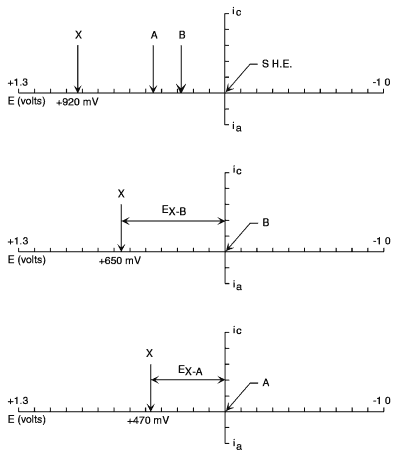Reference electrode A is situated at a potential more positive than that of reference electrode B. These potentials are dictated by variables such as the basic half-cell reaction, the concentration (formally the activities) of the participants in the half cell, temperature, etc. The potential axis upon which we have placed these two reference electrode half cells is a variable scale of oxidizing or reducing power. As we go to more positive potentials, the oxidizing power of the electrochemical detector increases; conversely, at more negative potentials, the power of the electrode to serve as a reducing agent improves. Suppose we impress between the reference and the working electrodes a potential difference of +0.5 V. As an example, let's take the compound caffeic acid, which oxidizes at approximately +500 mV with respect to the Ag/AgCl reference electrode. If we were to select a reference electrode whose potential relative to Ag/AgCl were 200 mV more positive than that of the Ag/AgCl couple, the applied potential required on the electrochemical controller to achieve equivalent oxidizing power would only be +300 mV. It is important to realize that the electrochemical potential axis is arbitrary in the sense that the reference electrode sets the zero point for this axis. For this reason, the reporting of electrochemical detector potentials must be referenced against the type of reference electrode used to complete the electrochemical cell. For example, it is commonplace to say caffeic acid oxidized at a potential of +500 mV vs. Ag/AgCl.

In the case of catastrophic failure, where the reference electrode potential might shift to a value of +500 mV with respect to its proper value, the performance of the electrochemical detector can be catastrophic as well. The electronic controller is still applying the same potential between the reference and working electrodes; it does not sense that a change in the reference electrode potential has taken place. Therefore, due to the shift, the actual oxidizing power of the working electrode has been increased to a sufficiently high level that objectionable and excessive oxidation of the mobile phase may occur (i.e., very large background current). You may not immediately discern whether the problem is due to the oxidation of some impurity in the mobile phase, deterioration of the working electrode surface, or a catastrophic change in the half-cell potential of the reference electrode couple. The problem must be diagnosed using a trial and error procedure where each of the above variables is tested independently of the others.

Since the half-cell potential of the reference electrode is taken as the arbitrary zero point along the electrochemical potential axis, it should not be surprising that oxidations may take place at negative potentials and reductions may take place at positive potentials. The positive or negative sign is merely an indication of where, in terms of absolute oxidizing or reducing power, the working electrode sits relative to the reference electrode. For example, if we selected a reference electrode whose potential was +1.0 V with respect to the present Ag/AgCl reference electrode potential, then nearly all electrochemical oxidations and reductions would be reported at negative potentials versus this new reference electrode. Specifically, in the case of pentachlorophenol whose oxidation potential vs. Ag/AgCl is approximately +800 mV, the new oxidation potential for an equivalent response would now be -200 mV because we would need to lower the working electrode's potential by that amount to make its oxidizing power equivalent to that when Ag/AgCl was used. For the reduction of a compound such as nitroglycerin where the applied potential vs. Ag/AgCl was -1.0 V, the new potential would be -2.0 V versus the new reference electrode.

Thus, we have transformed all of our oxidation and reduction potentials to a series of electrochemical potentials that are all negative, even though some of these are oxidation reactions where electrons will be passed into the electrode surface. Although it may seem contradictory that electrons would flow into an electrode with a negative potential, the issue here is not the charge on the electrode surface, but the establishment of a gradient of potential at the electrode/solution interface that is sufficient to cause the oxidation or reduction to occur. This difference in potential across the interface is what concerns the electrochemist! In all the cases we have described, the same interfacial potential difference would exist. A few more examples may be in order.

Let's take the case of the well-behaved electrochemical couple ferricyanide/ferrocyanide. This is a one-electron oxidation between two forms of iron held in a very stable hexacyano complex. Figure 1.10 demonstrates the oxidation and reduction of these complexes by cyclic voltammetry. Both reactions occur at positive applied potential.

Figure 1.10. Oxidation and reduction of iron hexacyano complex using cyclic voltammetry.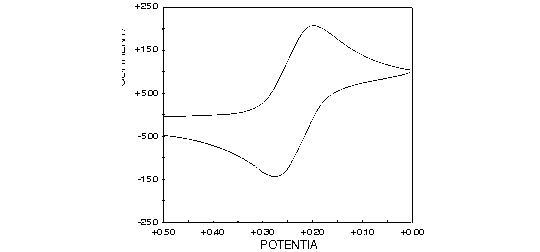Another application familiar to all electroanalytical chemists is anodic stripping voltammetry. In this technique, the potential of the working electrode—usually mercury or mercury-coated graphite—is held at a sufficiently negative potential, typically -1.0 V vs. Ag/AgCl, while trace metals are deposited to form a concentrated amalgam of metallic atoms in the zero oxidation state. After a sufficient deposition period, the potential is scanned in the positive direction, and the metal atoms are characteristically stripped from the mercury into solution via a forced oxidation to their ionic states. The potential of each of these stripping reactions occurs at a value characteristic of the metal ion being analyzed (Figure 1.11). Note that all of these oxidation reactions are done at negative potentials versus the reference electrode implied.

Figure 1.11. Anodic stripping voltammetry of trace metal ions using a BASi 100B/W Electrochemical Workstation and an Hg/Au voltammetry electrode. The stripping peaks correspond to the oxidation (all at negative potentials) of trace metals electrodeposited in the mercury.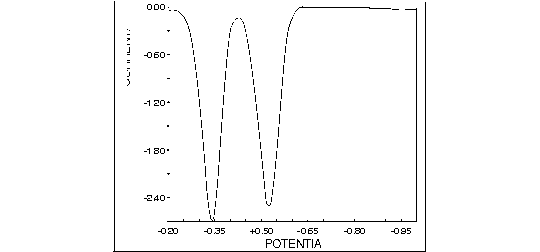In summary, we should make clear the following points about reference electrodes and their use:

1. The reference electrode serves as our electrochemical zero point on the applied potential axis, and for this reason, when potentials are reported either for cyclic voltammetry, LCEC, or other purposes, the reference electrode must be specified.

2. The potential of the reference electrode is determined by the half-cell couple involved. In the case of Ag/AgCl, the reference electrode potential is determined by the integrity of the Ag/AgCl wire in the electrode and the concentration of chloride ion in the reference electrode filling solution. The concentration of chloride ion in the reference electrode will necessarily change with time due to the concentration gradient across the reference electrode frit. This small change in the reference electrode potential may be retarded by careful storage of the reference electrode in 3 M sodium chloride. Alternately, the change may be compensated by a corresponding change in the potential applied to the cell by the electrochemical controller.

3. The sign of the applied potential is determined by the relative magnitude of the oxidizing or reducing power desired, relative to the reference electrode's potential. There are several types of reductions that may be carried out at positive potentials and an equally diverse number of oxidations that may be done at negative potentials with respect to the Ag/AgCl couple. Keep in mind that these sign conventions are relative and not fixed.

## MOBILE PHASE LIMITATIONS

Since amperometric detection depends on the transfer of electrons between a solute and the electrode surface, it is important to choose a solvent (mobile phase) that effectively permits the electrode reaction to occur. The primary limitations on the mobile phase are:

1. ELECTROLYTE MUST BE PRESENT, usually at 0.01 M to 0.1 M concentrations, to convey charge through the electrochemical cell.

2. The solvent must have a sufficiently high dielectric constant to freely permit IONIZATION OF THE ELECTROLYTE.

3. The mobile phase (electrolyte + solvent) must be ELECTROCHEMICALLY INERT at the electrode surface; that is, the background current at the applied potential should be negligible, with no chemical deterioration of the surface.

Even under the above restrictions, the scope of LCEC is very wide, since all ion-exchange and most reversed-phase separations employ these types of mobile phases. Initially all reports on LCEC dealt exclusively with ion-exchange columns using aqueous buffers. A majority of the separations performed with LCEC are now done with reversed-phase packing materials (e.g., BASi Biophase ODS or Phase II bonded phases). By bonding silyl hydrocarbon chains to a particle of silica, one fashions a packing capable of retaining nonpolar and weakly polar solutes from polar mobile phases. The versatility of reversed-phase materials can be further extended to ionic solutes by adding small amounts of ion pairing reagents to the mobile phase (e.g., BASi P/N CF-1090 sodium octyl sulfate). Polar bonded phases (diol or nitrile) have also been used. Few applications with silica gel columns have been reported, since the mobile phases needed to effect separations on these materials (nonpolar solvents with low dielectric constants) are incompatible with the requirements of LCEC. There are some ways to get around this problem with nonaqueous solvents.

## UNCOMPENSATED RESISTANCE

The simplest electronic equivalent of a three-electrode electrochemical cell would resemble Figure 1.12. The goal of the instrumentation is to impress all of the potential applied by the potentiostat across the interface between the electrode surface and the solution. This presents the sharpest potential gradient to the molecules.

Figure 1.12. Simple electronic equivalent of a detector cell.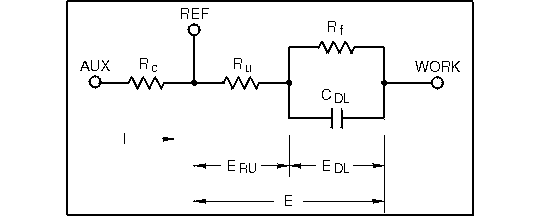Since the potential is applied between the reference and working electrodes, the user's control of the distribution of this potential between Ru and the interfacial double-layer impedance is impossible. The reference electrode may be moved closer to the working electrode (thereby minimizing Ru, but the term Ru will always be present to some degree. For this reason, Ru is termed the uncompensated resistance. CDL is the double-layer capacitance (this capacitance is charged up when the detector is turned on, and is the cause of the initial off-scale transient) while Rf is the faradaic resistance. The latter term represents the resistance to charge transfer across the interfacial region.

Since uncompensated resistance is always present, the simplest expression possible for the applied potential, E, is

E = ERU + EDL

Figure 1.12 describes this distribution. The difference between E and real applied potential EDL is the term ERU Since the electrical equivalent in this region is a simple resistor,

ERU = iRu

where i is the current passing through Ru (and the double layer) at any time. This factor, dependent on both the current and the resistance in the cell, is referred to as "iR drop."

The cells described in Figures 1.2 and 1.3 possess very low uncompensated resistance. The electrodes face each other directly across the thin-layer channel. Hence, even if cell currents reach hundreds of microamperes, ERU remains negligible and the interfacial potential remains constant.

The "curse" of high uncompensated resistance is narrow dynamic range. Spatial relationships within electrochemical cells are critical, and the compact nature of these two updated thin-layer designs is optimal in this regard.

## THE CURRENT RESPONSE

Two principal contributions to the current response of a thin-layer electrochemical cell are encountered. These are:

1. The FARADAIC RESPONSE, due to redox processes either from analyte or solvent impurities, and

2. The CHARGING CURRENT, required to charge the double-layer capacitance at the solution/electrode interface.

Normally, the charging current is not an issue since the detector is operated at fixed potential. Once the potential is supplied to the cell, and the background decays to an acceptably flat level, only faradaic contributions exist.

The fundamental relation for LCEC operation,

i = nFAJx=0

describes the current in terms of the flux J and various constants. The flux is dependent on the flow rate, cell dimensions, and the diffusion coefficient and concentration of the analyte being oxidized or reduced. A rigorous derivation incorporating a detailed expression for the flux permits the following equation:

ilim = 1.467 nFAC0(D/h2/3)(Uv/d)1/3

where n is the number of electron equivalents/mole, F is Faraday's constant, A is the area of the electrode (cm2), C0 is the concentration of reactant in the bulk eluent (mol/cm3), D is the diffusion coefficient (cm2/s), h is the thickness of the channel, U is the volume flow rate (cm3/s), and d is the width of the channel. From this expression, it is evident that the diffusion-limited current should be proportional to the concentration of the analyte, the area of the electrode, and the cube root of the velocity through the cell, and inversely related to the cube root of the channel thickness.